# Robustness of linear mixed models

Linear mixed models form an extremely flexible class of models for modelling continuous outcomes where data are collected longitudinally, are clustered, or more generally have some sort of dependency structure between observations. They involve modelling outcomes using a combination of so called fixed effects and random effects. Random effects allow for the possibility that one or more covariates have effects that vary from unit (cluster, subject) to unit. In the context of modelling longitudinal repeated measures data, popular linear mixed models include the random-intercepts and random-slopes models, which respectively allow each unit to have their own intercept or (intercept and) slope.

As implemented in statistical packages, linear mixed models assume that we have modelled the dependency structure correctly, and that both the random effects and within-unit residual errors follow normal distributions, and that these have constant variance. While it is possible to some extent to check these assumptions through various diagnostics, a natural concern is that if one or more assumptions do not hold, our inferences may be invalid. Fortunately it turns out that linear mixed models are robust to violations of some of their assumptions.

Modelling assumptions
Here we will follow developments of Verbeke and Molenberghs from their book ’Linear Mixed Models for Longitudinal Data’. We let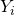denote the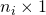vector of outcomes for unit. Of course what the unit corresponds to depends on the setting. For example in longitudinal studies of people each person is a unit. The outcomes of different units are assumed to be statistically independent of each other. The linear mixed model then assumes that: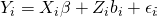The matrices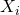and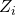are design which correspond to the fixed and random effects respectively. The vector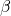describes the effect of covariates on the mean/expectation of the outcome, while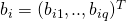is the vector random effects for unit. The random effects are assumed to be normally distributed with mean zero, and when there is more than one for each unit, some assumption on the correlation between the random effects must be made. Lastly,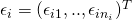is the vector of residual errors. We assume that these are normally distributed with variance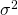, and that the errors within units are mutually independent.

An important point to note for the following is that the model implies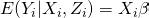under the assumption that we have correctly modelled how the mean ofdepends on the covariates, as coded in the design matrix.

Robustness results
The model parameters consists of the fixed effectsand the parameters involved in the variance/covariance matrix of the random effect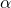. These are estimated by maximum likelihood or restricted maximum likelihood. These two approaches result in different estimators of the variance parameter, but for both the estimator ofis given by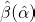, where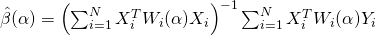where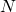denotes the number of units and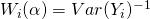, and we make clear that the dependence of the estimator on the estimated value of. Notice that this formula for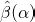is very close to the ordinary least squares estimator for the standard linear regression model, with the only difference being the presence here of the matrix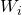.

Now if we take expectations ofconditional on the design matrices and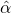, we have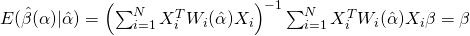We have thus shown that the estimator of the fixed effects parameteris unbiased, and the only assumption needed for this is that. This means that we obtain unbiased estimates even if our assumptions about random effects and residuals are inappropriate. For example, in modelling of repeated measures with a random intercepts and slopes model, we assume that each unit’s underlying trajectory is linear, with the intercept and slope specific to each unit. If in truth the unit’s trajectories are not linear, we will obtain unbiased estimates of(assuming we have correctly specified the fixed effects part).

Alternatively, the random effects and/or residual errors might not be normally distributed. Nonetheless, we continue to obtain unbiased estimates of. The result also holds even if the variances (of random effects or residual errors) are not constant. For example, in a study including men and women, the random-effects might have different variances for men and women. Even if this heteroscedascity is not modelled, we still obtain unbiased estimates of, although we will lose some efficiency.

Effects on inferences and link to GEEs
Although the fixed effects might be estimated without bias under non-normality or heteroscedascity, we must be careful when drawing inferences – i.e. estimating standard errors, and calculating p-values and confidence intervals. To understand the impacts on validity of inferences for, as described by ’Verbeke and Molenberghs’ (section 6.2.4) we can exploit the connection between linear mixed models and generalized estimating equations (GEEs) (see here for Liang and Zeger’s original paper on GEEs). Specifically, the likelihood score equations which are used to estimatecan be viewed as a GEE, with the correlation structure in the GEE corresponding to that implied by our choice of random effects in the linear mixed models.

First let’s suppose that we believe that our linear mixed model correctly models the correlation structure of the data, i.e. that our decomposition of within-unit dependence into that induced by the random effects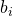and residual errors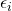is correct. However, we might not be entirely confident that the normality assumptions hold for the random effects and residual errors. In this case, the standard linear mixed model standard errors are still consistent, and consequently asymptotically our p-values and confidence intervals for the fixed effects will be valid. With longitudinal or clustered data ‘asymptotics’ are more complicated than in the non-clustered setting. For example with two level data (observations nested within units), we need the number of units as well as the observations to be ‘large’ for the asymptotic results to be applicable.

Next, suppose that we are not even confident that we have correctly modelled the correlation structure of the data. In this case the usual standard errors calculated by our linear mixed model commands will not be consistent. As a consequence, our p-values and confidence intervals may be invalidated. One of the important developments in Liang and Zeger’s 1986 paper on GEEs was the so called robust sandwich variance estimator. The sandwich variance estimator is consistent even if we have misspecified the correlation structure. What does this mean in practice? If we are not confident that we have modelled the correlation structure in our data correctly in the linear mixed model, we should switch to using a GEE and make use of the robust sandwich variance estimator.

Software
In R the geepack package can be used to fit a GEE and the robust sandwich variance estimator is the default option. In Stata, the xtgee can similarly be used, but note that in this case the vce(robust) option must be used. In fact, Stata’s linear mixed model command mixed actually allows the vce(robust) option to be used.

A small simulation study
We can perform a small simulation study to illustrate the preceding results. The R code below generates datasets for 100 units, with each unit having 5 measurements. Measurement times are generated for each unit from a uniform distribution on the range 0-10. A random intercept and slope is then generated for each unit from a chi squared (i.e. not normal) distribution. The outcomes are then generated from the model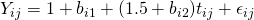where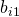and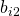is unit i’s random intercept and slope and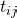is the time of measurement for the j’th measurement on unit i. Here we generate the residual errors from a normal distribution, but the random effects have a skewed, non-normal distribution. The true value of the fixed effects parameter is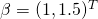. We use the lme4 package to fit the random intercepts and slopes model

library(lme4)

nsim <- 1000
fixedEffEst <- array(0, dim=c(nsim,2))
fixedEffSE <- array(0, dim=c(nsim,2))

n <- 100
n_i <- 5
id <- rep((1:n),n_i)

for (i in 1:nsim) {
print(i)
times <- array(10*runif(n*n_i), dim=c(n*n_i,1))

randomIntercept <- rep(rnorm(n)^2-1, n_i)
randomSlope <- rep(2*rnorm(n)^2-2, n_i)

residualerror <- rnorm(n*n_i)
y <- 1+randomIntercept+(1.5+randomSlope)*times+residualerror

lmm <- lmer(y~times+(1+times|id))
fixedEffEst[i,] <- fixef(lmm)
fixedEffSE[i,] <- summary(lmm)\$coefficients[1:2,2]

}

colMeans(fixedEffEst)
sd(fixedEffEst[,1])
sd(fixedEffEst[,2])
colMeans(fixedEffSE)

#CI coverage
interceptCI <- cbind(fixedEffEst[,1]-1.96*fixedEffSE[,1],fixedEffEst[,1]+1.96*fixedEffSE[,1])
mean((interceptCI[,1]<1) & (interceptCI[,2]>1))
slopeCI <- cbind(fixedEffEst[,2]-1.96*fixedEffSE[,2],fixedEffEst[,2]+1.96*fixedEffSE[,2])
mean((slopeCI[,1]<(1.5)) & (slopeCI[,2]>(1.5)))


In the final part of the code we obtain the mean of the estimates of the fixed effects estimates and the standard deviation of the estimates across 1,000 simulations. We then construct 95% Wald confidence intervals for the two components of, and examine their coverage over the 1,000 simulations. Upon running I obtained:

> colMeans(fixedEffEst)
 0.9985826 1.5068368
> sd(fixedEffEst[,1])
 0.1757847
> sd(fixedEffEst[,2])
 0.2814766
> colMeans(fixedEffSE)
 0.1740634 0.2796163
>
> #CI coverage
> interceptCI <- cbind(fixedEffEst[,1]-1.96*fixedEffSE[,1],fixedEffEst[,1]+1.96*fixedEffSE[,1])
> mean((interceptCI[,1]<1) & (interceptCI[,2]>1))
 0.951
> slopeCI <- cbind(fixedEffEst[,2]-1.96*fixedEffSE[,2],fixedEffEst[,2]+1.96*fixedEffSE[,2])
> mean((slopeCI[,1]<(1.5)) & (slopeCI[,2]>(1.5)))
 0.926


As the theory predicts, we see that the fixed effect estimates are unbiased (the means are close to 1 and 1.5 for the two parameters). The average of the estimated standard errors are also close to the empirical standard deviation of the estimates. The 95% CI of the intercept has coverage almost identical to 95%. The interval for the slope is slightly below the nominal 95% level.

Here we were able to obtain valid inferences using the standard linear mixed model command and standard model based standard errors because the linear mixed model we fitted correctly modelled the correlation structure. That is, the data were generated from a random intercepts and slopes model, and we fitted a random intercepts and slopes model. The fact that the random effects were not normally distributed did not adversely affect the validity of inferences. If instead we were not confident in the random intercepts and slopes assumption, we should switch to fitting a GEE with the robust sandwich variance estimator.

Caveats
Here we have focused on inference on the fixed effects parameter, and have seen that inferences can be robust to various violations of modelling assumptions. However, often we are of course not only interested in the fixed effects but also in the random effects, which describe how the multiple measurements on units are correlated after taking the fixed effects into account. In this case the modelling assumptions made by the linear mixed model are essential. Moreover, correctly modelling the correlation structure of the data results in more efficient estimates of.

A further important caveat is when our dataset suffers from missing outcome values. When some outcomes are missing, linear mixed models provide valid inferences under the so called missing at random assumption. However, this statement is contingent on the modelling assumptions made being correct for the data at hand. Consequently when we have missing data, correctly modelling the correlation structure is essential even when we are only interested in the fixed effects parameter.

For more on the topic of longitudinal/clustered data analysis of continuous data, I’d highly recommend ’Linear Mixed Models for Longitudinal Data’ by Verbeke and Molenberghs. They also have a corresponding book for the analysis of longitudinal/clustered discrete data: ’Models for Discrete Longitudinal Data’.

### 4 thoughts on “Robustness of linear mixed models”

1.Thank you, this helped a lot as Identification assumptions of linear mixed models are often not clearly presented.

2.Same here: Your clarity is much appreciated.

3.•